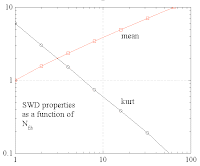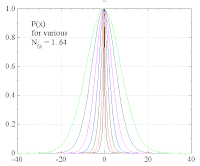## Thursday, June 12, 2008

### Apollon-Project: Bead and Fiber SWD

How is the SWD (form of distribution, mean value Dm, kurtosis kur) of the bead related to the SWD of the individual fibers ?

Assumptions:
• Spider geometry with N_fib fibers.
• Individual fibers have identical exponential SWDs with average step width dMean.
Program: Apollon_1

Parameters: dMean=1.0

Results:
• dMean acts as a scaling parameter.
• NFib acts as a shape parameter.
• sqrt(NFib) ~ Dm ~ dMean
• 1/Nfib ~ kur <--indep--> dMeanAs for the SWD itself, one can either analyze the distributions P(x), P(y) of the cartesian components of the bead displacement, or the radial distribution P(r).

In the case of P(x), one finds as a function of N_fib a gradual evolution from exponential to Gaussian:The radial distribution P(r) corresponds, of course, to xP(x) and approaches in the limit of infinitely many fibers a Rayleigh distribution:So far, the well stiffness kWell was a constant. What happens to the above results when kWell grows in proportion to the number of myosins NMyo in the system ? NMyo is proportional to the number of fibers NFib and to the average fiber size fMean, which depends in turn on the remodelling rate |df/dt| (fibers grow to higher peak sizes within the same time).

With myosin-depending stiffness, KWell ~ NMyo ~ NFib. Since dMean ~ 1/kWell, we get an extra factor dMean ~ 1/NFib. Together with the factor dMean ~ sqrt(NFib) we arrive at

dMean ~ 1/sqrt(NFib).

Note that dMean determines the overall diffusion constant D of the MSD. So with myosin-depending stiffness, D will decrease with the number of fibers. With constant stiffness it will increase. The experiments favor myosin-dependent stiffness.

Note also that kWell does not change the shape of the SWD, only the scale.

It is possible to reasonably fit the SWD to experimental data, at least within the main peak regime:There is a certain flexibility: a higher N_fib can be approximately compensated by a smaller dMean.